# Labop Datasets

19 Mar 2023 - Daniel Bryce (danbryce.bioprotocols@gmail.com)

The Bioprotocols Working Group is pleased to announce a new LabOP feature for handling dataset specifications within prototcols.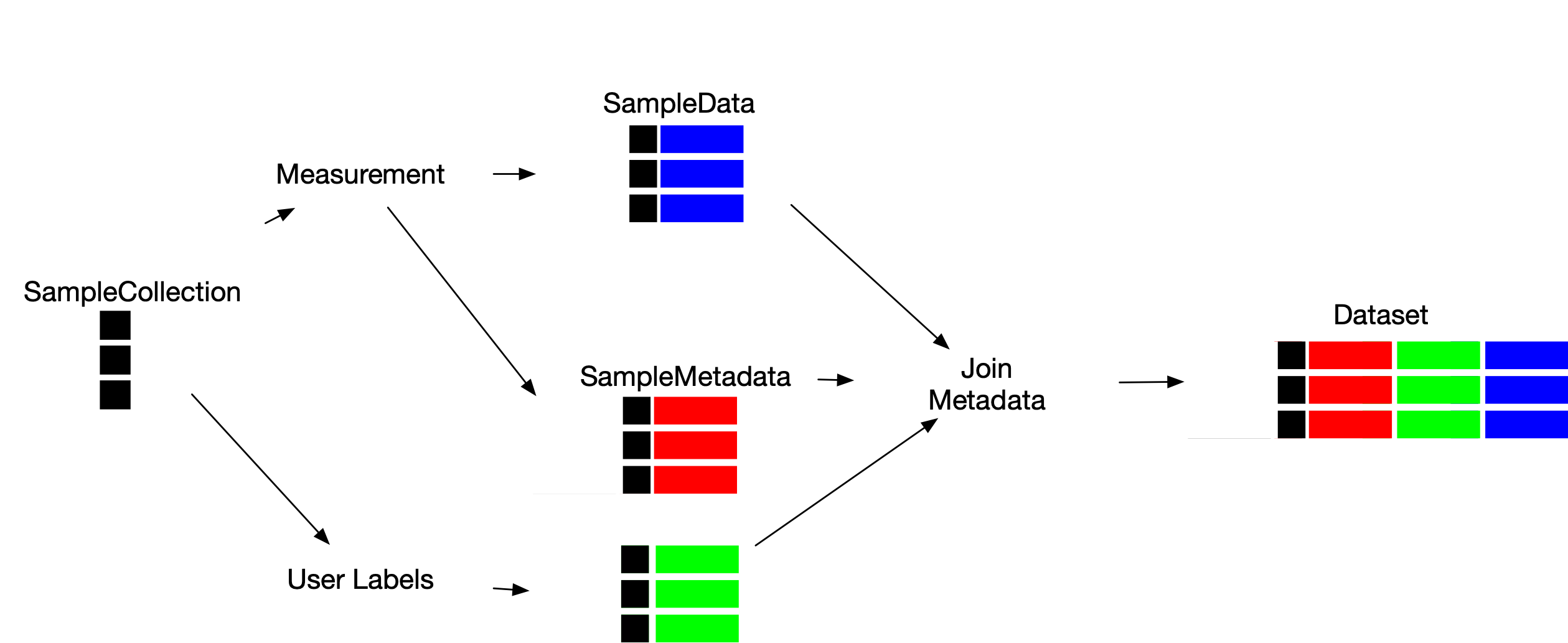This new feature benefits LabOP users, as follows:

• Prescribe experimental data to collect, and incorporate data values after collection,
• Link experimental data and metadata to reproducibly consolidate a dataset specification, and
• Support dataset development through programmatic interfaces to devices or manual data entry.

# Terminology

LabOP Datasets represent the data and metadata for sample measurements. LabOP protocols prepare datasets by linking several data types, as follows:

• `SampleCollection`: representing a collection of samples, with two subtypes:
• `SampleArray`: representing an array of sample identifiers
• `SampleMask`: representing a subset of samples from a `SampleCollection`
• `SampleData`: representing data for each sample in a referenced `SampleCollection`
• `SampleMetadata`: representing metadata for each sample in a referenced `SampleCollection`
• `Dataset`: representing a collection of `SampleData`, `SampleMetadata`, and `Dataset` objects

A `Dataset` thus consolidates data and metadata for samples. A LabOP protocol describes how to construct a `Dataset` through multiple primitives, as follows:

1. Sample Creation: several primitives (e.g., EmptyContainer, PlateCoordinates, etc.) output a `SampleCollection` that describes several samples.
2. Metadata Generation: primitives (e.g., ExcelMetadata) either output `Metadata` directly, or indirectly (e.g., MeasureAbsorbance) as part of a `Dataset` output.
3. Sample Data Generation: primitives (e.g., MeasureAbsorbance) also indirectly generate `SampleData` (referencing a `SampleCollection`) as part of generating their `Dataset` output
4. Dataset Generation: as noted above, measurement primitives (e.g., MeasureAbsorbance) generate `Dataset` output
5. Dataset Consolidation: the JoinMetadata primitive creates a `Dataset` from a `SampleMetadata` and `Dataset`, and the JoinDataset primitive creates a `Dataset` from one or more `Dataset` and an optional `SampleMetadata`.

# LabOP Protocol Dataset Specification Example

The following example illustrates how a protocol can construct a `Dataset` that includes multiple `SampleMetadata`. The protocol involves measuring the absorbance of a subset of samples, and developing metadata that describes both the sample contents and measurement instrument parameters.

The figure below illustrates the complete protocol. In the figure, we illustrate each primitive by three layers of rectangles, where rectangles in the top row are input ports, rectangles on the bottom row are output ports, and the center rectangle is the primitive name. The blue edges denote control flow ordering, and black edges denote data flow. The rectangle with a double border is an output parameter. The black circle is the initial node, and the thin black rectangle is a fork node (indicating data flow from a single source to multiple targets).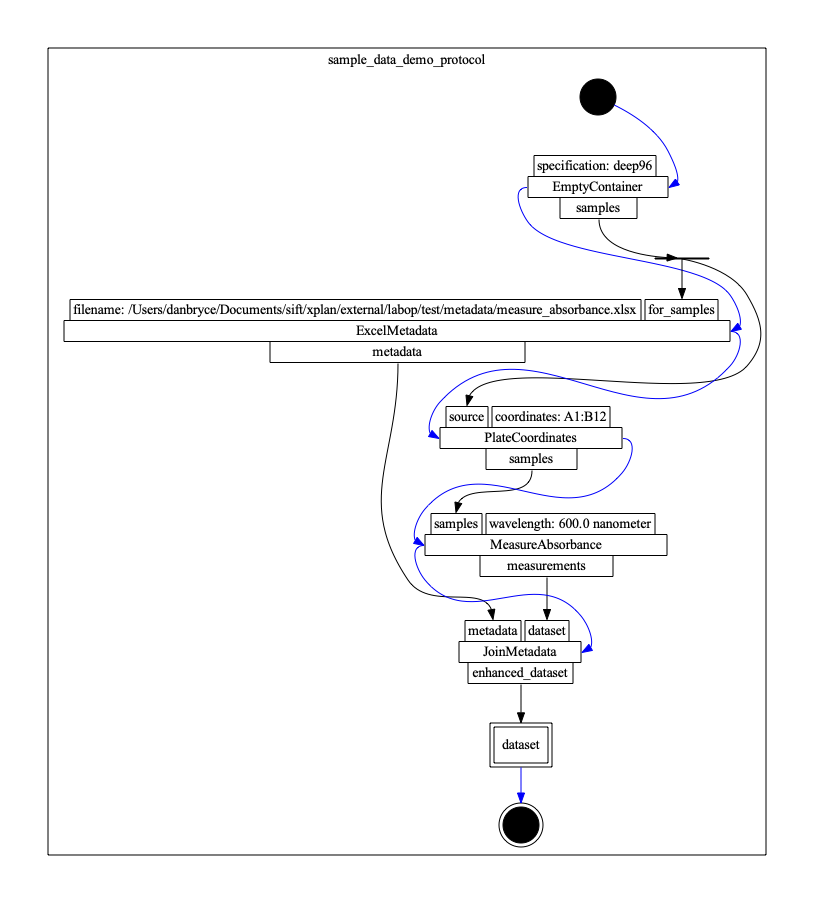The protocol involves creating a `SampleArray`, indicating a subset of samples to measure, measuring the samples, reading predefined `SampleMetadata`, and joining metadata to create a `Dataset`. We detail the protocol definition using the LabOP python library below.

1. Initialize Protocol:

The following code block initializes an empty `Protocol` and `sbol3.Document`.

``````Import os
from tyto import OM
import sbol3
import labop
from labop.utils.helpers import initialize_protocol

protocol, doc = initialize_protocol()
``````
2. Sample Creation:

Creating a `SampleCollection` starts with the `EmptyContainer` primitive, and a `ContainerSpec`.

``````# Define the type of container
container_type = container_type = labop.ContainerSpec('deep96')

# Create an activity to create the container.
create_source = protocol.primitive_step(
'EmptyContainer’,
specification=container_type
)
``````

The `samples` output pin for the `create_source` object (of type `CallBehaviorExecution`) is a `SampleArray` object whose `samples` attribute will (at runtime) be assigned a serialized xarray DataArray object of the form:

``````<xarray.DataArray 'https://bioprotocols.org/demo/test_execution/ActivityEdgeFlow3/LiteralIdentified1/SampleArray1' (
sample: 96)>
array(['A1', 'B1', 'C1', 'D1', 'E1', 'F1', 'G1', 'H1', 'A2', 'B2', 'C2',
'D2', 'E2', 'F2', 'G2', 'H2', 'A3', 'B3', 'C3', 'D3', 'E3', 'F3',
'G3', 'H3', 'A4', 'B4', 'C4', 'D4', 'E4', 'F4', 'G4', 'H4', 'A5',
'B5', 'C5', 'D5', 'E5', 'F5', 'G5', 'H5', 'A6', 'B6', 'C6', 'D6',
'E6', 'F6', 'G6', 'H6', 'A7', 'B7', 'C7', 'D7', 'E7', 'F7', 'G7',
'H7', 'A8', 'B8', 'C8', 'D8', 'E8', 'F8', 'G8', 'H8', 'A9', 'B9',
'C9', 'D9', 'E9', 'F9', 'G9', 'H9', 'A10', 'B10', 'C10', 'D10',
'E10', 'F10', 'G10', 'H10', 'A11', 'B11', 'C11', 'D11', 'E11',
'F11', 'G11', 'H11', 'A12', 'B12', 'C12', 'D12', 'E12', 'F12',
'G12', 'H12'], dtype='<U3')
Coordinates:
* sample   (sample) <U3 'A1' 'B1' 'C1' 'D1' 'E1' ... 'E12' 'F12' 'G12' 'H12'
``````

The `ExcelMetadata` primitive creates a `SampleMetadata` object from the contents of an xlsx file with a workbook depicted in the following image: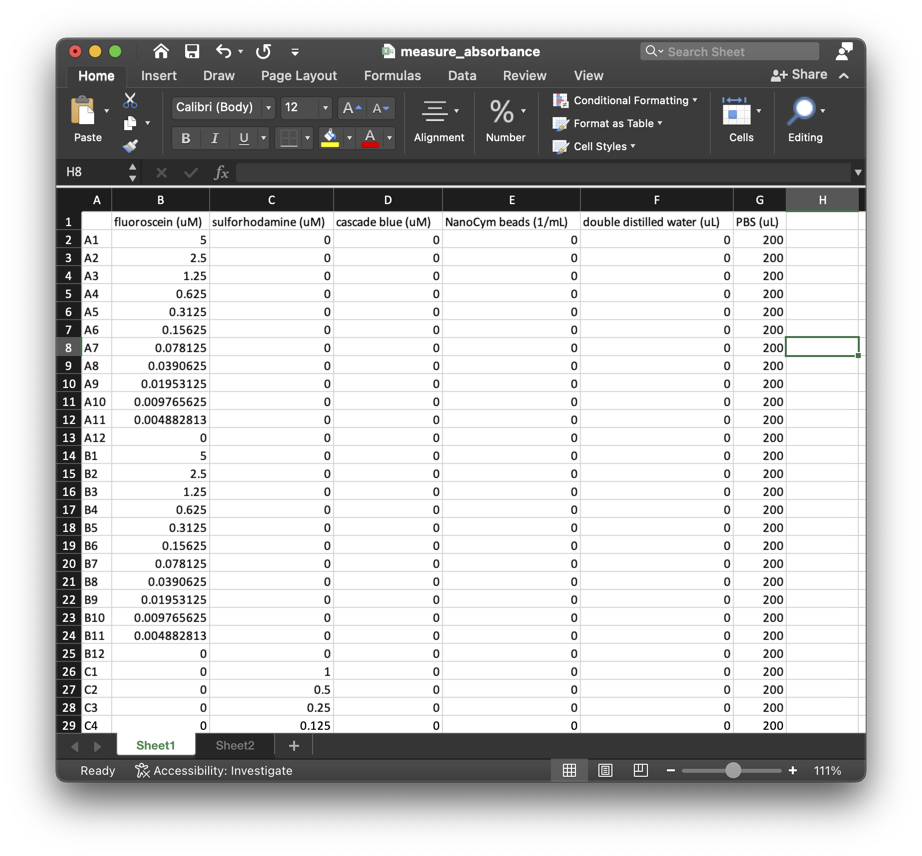The `SampleArray` created by the `EmptyContainer` primitive includes references to samples that appear in the xlsx file.

``````filename = os.path.join(os.getcwd(), "test", "metadata", "measure_absorbance.xslx”)

for_samples=create_source.output_pin('samples'),
filename=filename
)
``````

The `SampleMetadata` object created by executing the `ExcelMetadata` primitive has a serialized xarray Dataset of the form:

``````<xarray.Dataset>
Dimensions:                      (sample: 96)
Coordinates:
* sample                       (sample) <U3 'A1' 'A2' 'A3' ... 'H11' 'H12'
Data variables:
fluorescein (uM)             (sample) float64 5.0 2.5 1.25 ... 0.0 0.0 0.0
sulforhodamine (uM)          (sample) float64 0.0 0.0 0.0 ... 0.0 0.0 0.0
cascade blue (uM)            (sample) float64 0.0 0.0 0.0 ... 0.0 0.0 0.0
NanoCym beads (1/mL)         (sample) float64 0.0 0.0 ... 7.324e+05
double distilled water (uL)  (sample) int64 0 0 0 0 0 ... 200 200 200 200
PBS (uL)                     (sample) int64 200 200 200 200 200 ... 0 0 0 0
``````

The final `Dataset` defines the value of several metadata attributes (variables) for each sample, originally appearing as columns in the xlsx file.

4. Measurement Data and Metadata Generation:

The `MeasureAbsorbance` primitive reports a `Dataset` with `SampleMetadata` and `SampleData` for the `SampleCollection` defined by its `samples` attribute. In this example, the `SampleCollection` is a `SampleMask` that identifies a subset of the samples as an xarray DataArray:

``````create_coordinates = protocol.primitive_step(
'PlateCoordinates',
source=create_source.output_pin('samples'),
coordinates="A1:B12”
)
``````

The `samples` output pin represents the mask as an xarray DataArray of the form:

``````<xarray.DataArray 'https://bioprotocols.org/demo/test_execution/ActivityEdgeFlow8/LiteralIdentified1/SampleMask1' (
sample: 24)>
array(['A1', 'B1', 'A2', 'B2', 'A3', 'B3', 'A4', 'B4', 'A5', 'B5', 'A6',
'B6', 'A7', 'B7', 'A8', 'B8', 'A9', 'B9', 'A10', 'B10', 'A11',
'B11', 'A12', 'B12'], dtype='<U3')
Coordinates:
* sample   (sample) <U3 'A1' 'B1' 'A2' 'B2' 'A3' ... 'A11' 'B11' 'A12' ‘B12'
``````

Executing `MeasureAbsorbance` produces a `Dataset` that relates a `SampleMetadata` and a `SampleData` object.

``````measure_absorbance = protocol.primitive_step(
'MeasureAbsorbance',
samples=create_coordinates.output_pin('samples'),
wavelength=sbol3.Measure(600, OM.nanometer)
)
``````

The `SampleData` holds the data for each sample with an xarray DataArray of the form:

``````<xarray.DataArray (sample: 24)>
array([nan, nan, nan, nan, nan, nan, nan, nan, nan, nan, nan, nan, nan,
nan, nan, nan, nan, nan, nan, nan, nan, nan, nan, nan])
Coordinates:
* sample   (sample) <U3 'A1' 'A2' 'A3' 'A4' 'A5' ... 'B9' 'B10' 'B11' 'B12'
``````

The generated `SampleMetadata` includes information about the `MeasureAbsorbance` inputs (i.e., wavelength), as follows:

`````` <xarray.Dataset>
Dimensions:     (sample: 24)
Coordinates:
* sample      (sample) <U3 'A1' 'A2' 'A3' 'A4' 'A5' ... 'B9' 'B10' 'B11' 'B12'
Data variables:
wavelength  (sample) <U102 'https://bioprotocols.org/demo/demo_protocool/...
``````

The wavelength variable refers to a LabOP object that defines the wavelength as an ontologies of measure object that can be exported in a human readable format as “600 nanometer”.

5. Dataset Generation:

The `Dataset.to_dataset()` function can merge the xarray representations of the `SampleMetadata` and `SampleData` (i.e., join along the sample coordinates) to produce an xarray Dataset of the form:

``````<xarray.Dataset>
Dimensions:                                                                                                  (
sample: 24)
Coordinates:
* sample                                                                                                   (sample) <U3 ...
Data variables:
https://bioprotocols.org/demo/test_execution/ActivityEdgeFlow12/LiteralIdentified1/Dataset1/SampleData1  (sample) float64 ...
wavelength                                                                                               (sample) <U102 ...
``````

where `https://bioprotocols.org/demo/test_execution/ActivityEdgeFlow12/LiteralIdentified1/Dataset1/SampleData1` is data for each sample, and the `wavelength` is metadata for each sample. This intermediate `Dataset` relates the measurement `SampleMetadata` to the `SampleData`. In the following, we elaborate this `Dataset` with additional metadata.

1. Dataset Consolidation:

Finally, the `JoinMetadata` primitive can relate additional `SampleMetadata` to `Dataset` objects. In the following, the `JoinMetadata` primitive adds the sample metadata extracted from the xlsx file to the Dataset produced by the `MeasureAbsorbance` primitive:

``````meta1 = protocol.primitive_step(
dataset=measure_absorbance.output_pin('measurements'),
)
``````

The protocol returns a `dataset` parameter that reports the `enhanced_metadata` produced by `JoinMetadata` (including both sample descriptions and instrument settings, as well as the measurement data):

``````protocol.designate_output(
'dataset',
'http://bioprotocols.org/labop#Dataset',
source=meta1.output_pin('enhanced_dataset')
)
``````

The `Dataset.to_dataset()` function produces a consolidated xarray Dataset of the form:

``````<xarray.Dataset>
Dimensions:                                                                                                  (
sample: 96)
Coordinates:
* sample                                                                                                   (sample) <U3 ...
Data variables:
https://bioprotocols.org/demo/[...]/SampleData1  (sample) float64 ...
wavelength                                                                                               (sample) object ...
fluorescein (uM)                                                                                         (sample) float64 ...
sulforhodamine (uM)                                                                                      (sample) float64 ...
cascade blue (uM)                                                                                        (sample) float64 ...
NanoCym beads (1/mL)                                                                                     (sample) float64 ...
double distilled water (uL)                                                                              (sample) int64 ...
PBS (uL)                                                                                                 (sample) int64 ...
``````

The xarray Dataset can be converted to a number of formats, including a pandas Dataframe:

``````        https://bioprotocols.org/demo/[...]/Dataset1/SampleData1                                         wavelength  fluorescein (uM)  ...  NanoCym beads (1/mL)  double distilled water (uL)  PBS (uL)
sample                                                                                                                                                                                ...
A1                                               0.994238                                                        https://bioprotocols.org/demo/...          5.000000  ...                   0.0                            0       200
A10                                              0.690957                                                        https://bioprotocols.org/demo/...          0.009766  ...                   0.0                            0       200
A11                                              0.379377                                                        https://bioprotocols.org/demo/...          0.004883  ...                   0.0                            0       200
A12                                              0.006668                                                        https://bioprotocols.org/demo/...          0.000000  ...                   0.0                            0       200
A2                                               0.076588                                                        https://bioprotocols.org/demo/...          2.500000  ...                   0.0                            0       200
...                                                   ...                                                                                                      ...               ...  ...                   ...                          ...       ...
H5                                                    NaN                                                                                                      NaN          0.000000  ...            93750000.0                          200         0
H6                                                    NaN                                                                                                      NaN          0.000000  ...            46875000.0                          200         0
H7                                                    NaN                                                                                                      NaN          0.000000  ...            23437500.0                          200         0
H8                                                    NaN                                                                                                      NaN          0.000000  ...            11718750.0                          200         0
H9                                                    NaN                                                                                                      NaN          0.000000  ...             5859375.0                          200         0

[96 rows x 8 columns]
``````

or a more human readable format with `Dataset.humanize()`:

``````<xarray.Dataset>
Dimensions:                            (sample: 96)
Coordinates:
* sample                             (sample) <U3 'A1' 'A10' ... 'H8' 'H9'
Data variables:
MeasureAbsorbance.measurements.76  (sample) float64 nan nan nan ... nan nan
wavelength                         (sample) <U15 '600.0 nanometer' ... 'nan'
fluorescein (uM)                   (sample) float64 5.0 0.009766 ... 0.0 0.0
sulforhodamine (uM)                (sample) float64 0.0 0.0 0.0 ... 0.0 0.0
cascade blue (uM)                  (sample) float64 0.0 0.0 0.0 ... 0.0 0.0
NanoCym beads (1/mL)               (sample) float64 0.0 0.0 ... 5.859e+06
double distilled water (uL)        (sample) int64 0 0 0 0 ... 200 200 200
PBS (uL)                           (sample) int64 200 200 200 200 ... 0 0 0
``````

The execution engine also constructs an xlsx file that includes all `SampleData` and `Dataset` objects. The following figures illustrate two respective sheets of the xlsx workbook corresponding to the `SampleData` and the `Dataset`.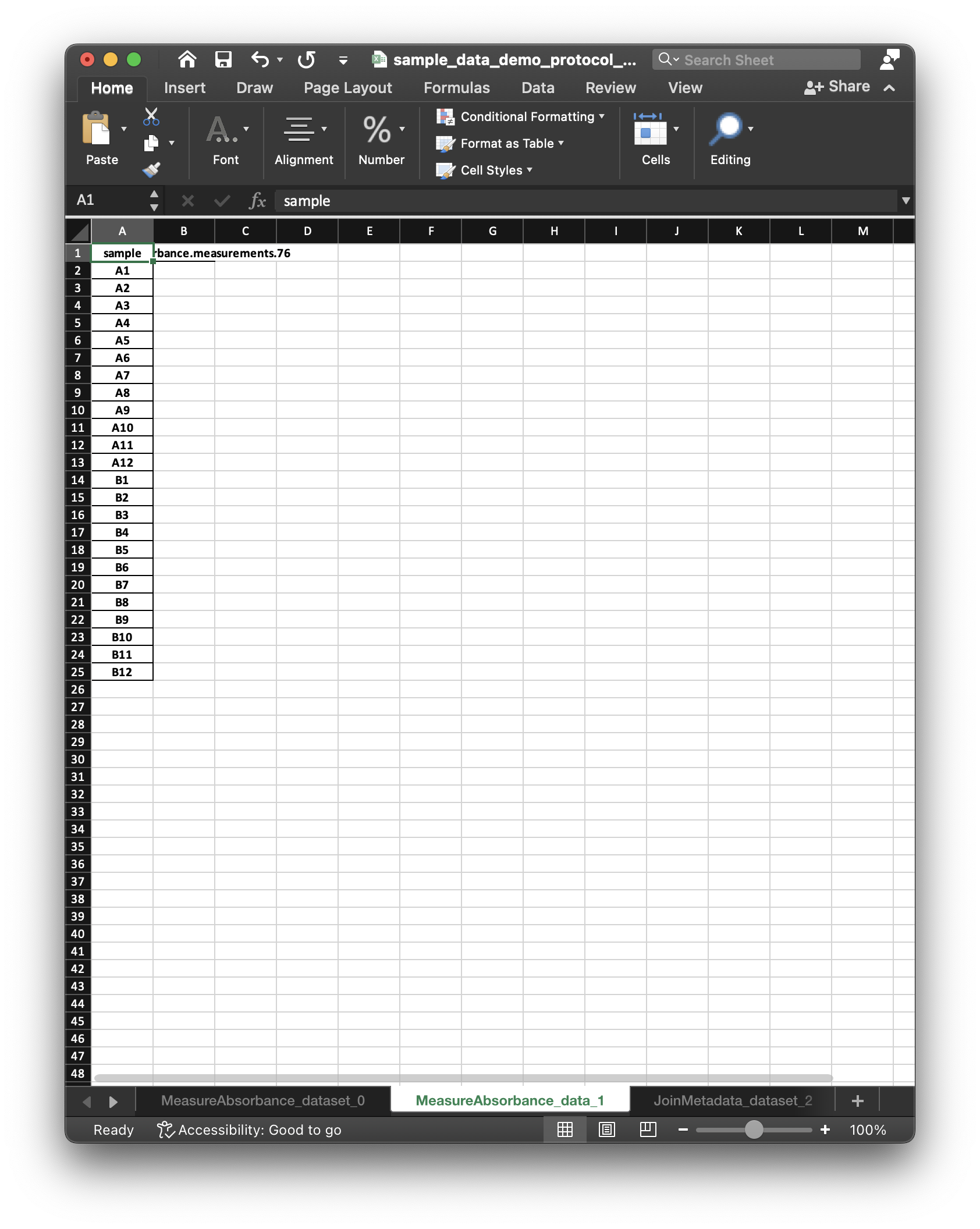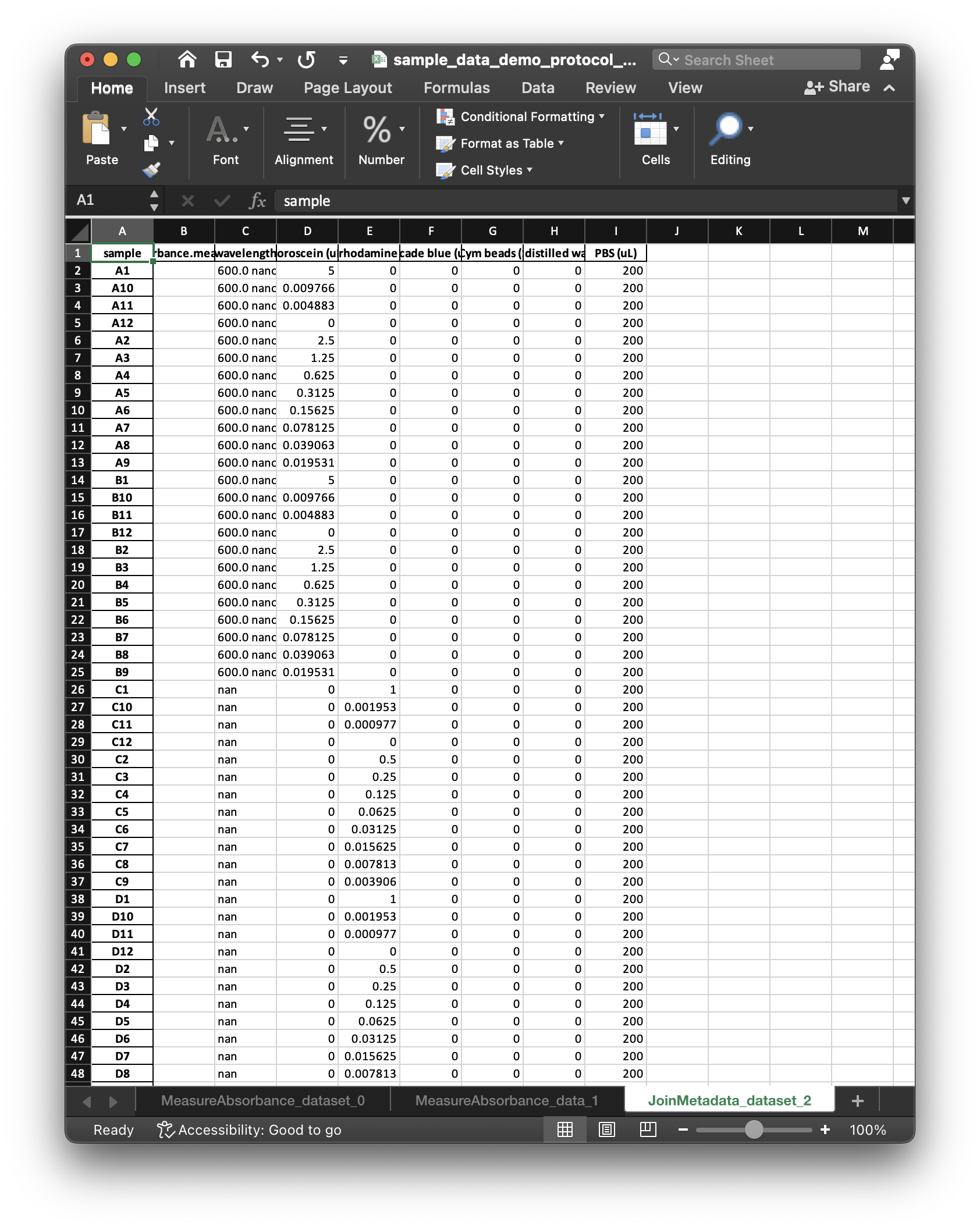The `SampleData` and `Dataset` worksheets include an empty column for the data to be collected, and (along with the protocol) correspond to what we mean by a “dataset specification”. After entering data values in the `SampleData` worksheet, LabOP can read the workbook to populate the `Dataset` with the entered values.

# Multicolor Calibration Protocol

As a full example, the multicolor calibration protocol uses similar dataset specification primitives to describe the `Dataset` comprising three types of fluorescence measurements and one type of absorbance measurement. LabOP creates several artifacts for the protocol, including:

• Markdown Document (md): the markdown representation of the protocol is a human readable document generated from the LabOP protocol by the LabOP library.
• Markdown Document (pdf): the pdf rendering of the markdown document includes additional linked figures.
• Dataset Workbook (xlsx): The Dataset workbook is generated by the LabOP library to describe the `SampleData` templates and the `Dataset` specification.
• Protocol Script: the python script will generate all protocol artifacts by using the LabOP library and execution engine to instantiate and perform “pre-flight” execution of the protocol.
• Protocol Visualization (pdf): The graphviz rendering of the protocol illustrates the activities required to execute the protocol in a UML-like format.
• Protocol Input Metadata: the input metadata describes the contents of the samples measured by the protocol, and is used to construct the `Dataset`.
• Protocol and Protocol Execution Trace RDF (nt): the RDF representation of the protocol and execution trace is the LabOP representation of the protocol that is used as the common protocol representation.